# Coupon Rate

It is the interest rate paid on a bond, stated as a percentage of the face value, also known as the par value or principal, of that bond.

The coupon rate is the interest rate paid on a bond, stated as a percentage of the face value, also known as the par value or principal, of that bond.

This is a fixed rate, giving bonds the term fixed income. Co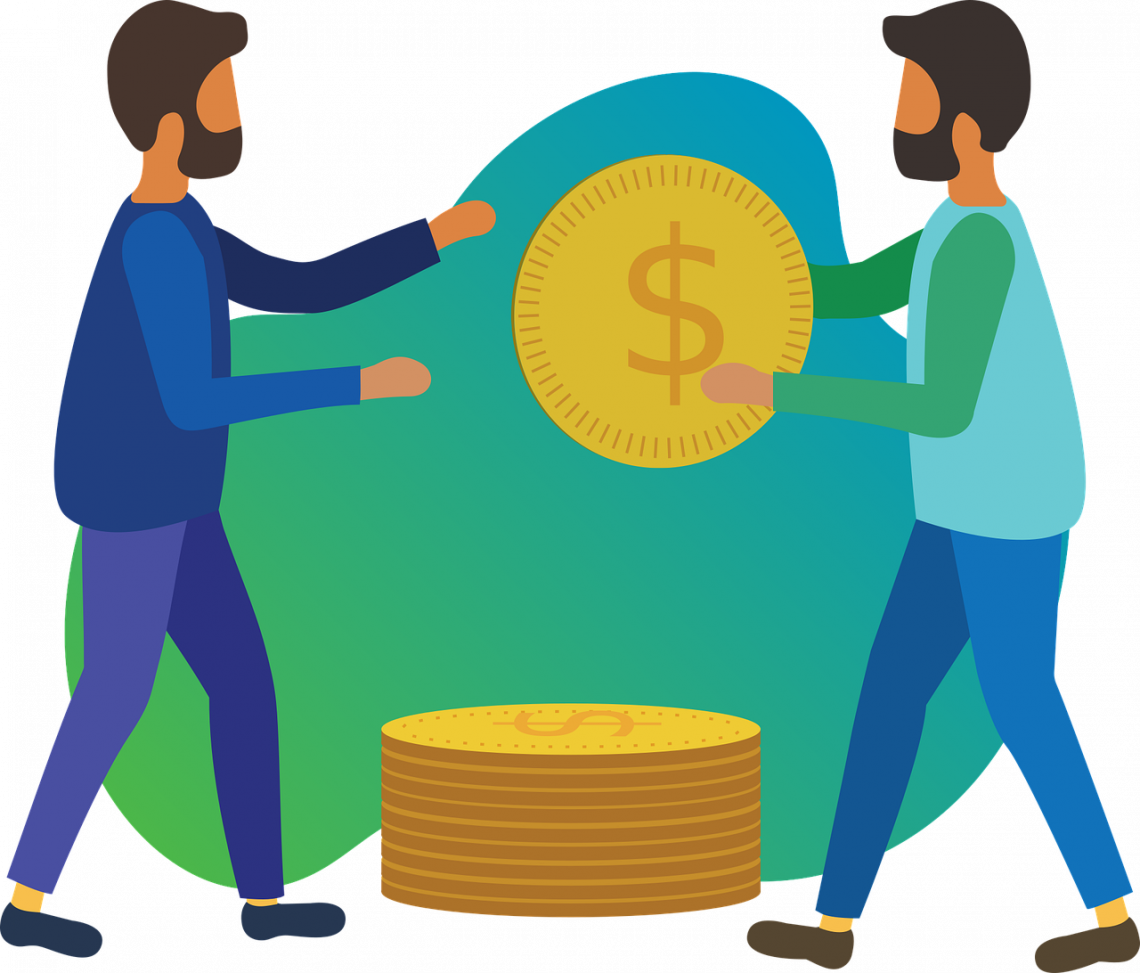companies and government entities issue bonds to fund funding and other interests.

This bond is a type of loan and is similar to other loans. The debtor compensates people for lending them money by paying them interest and returning the principal amount to them later.

The amount of interest that is paid out is considered the coupon payment.

In the case of bonds, the principal is not repaid until maturity. Every year, however, the lender receives coupon payments.

The term coupon comes from when coupons were redeemed interest on bonds.

## How to Calculate?

The coupon rate is simply a percentage of the bond's principal amount, which is also the face value of the bond.

The formula for finding this rate can be found below:

Coupon Rate = (Annual Coupon Payment / Bond Face Value) * 100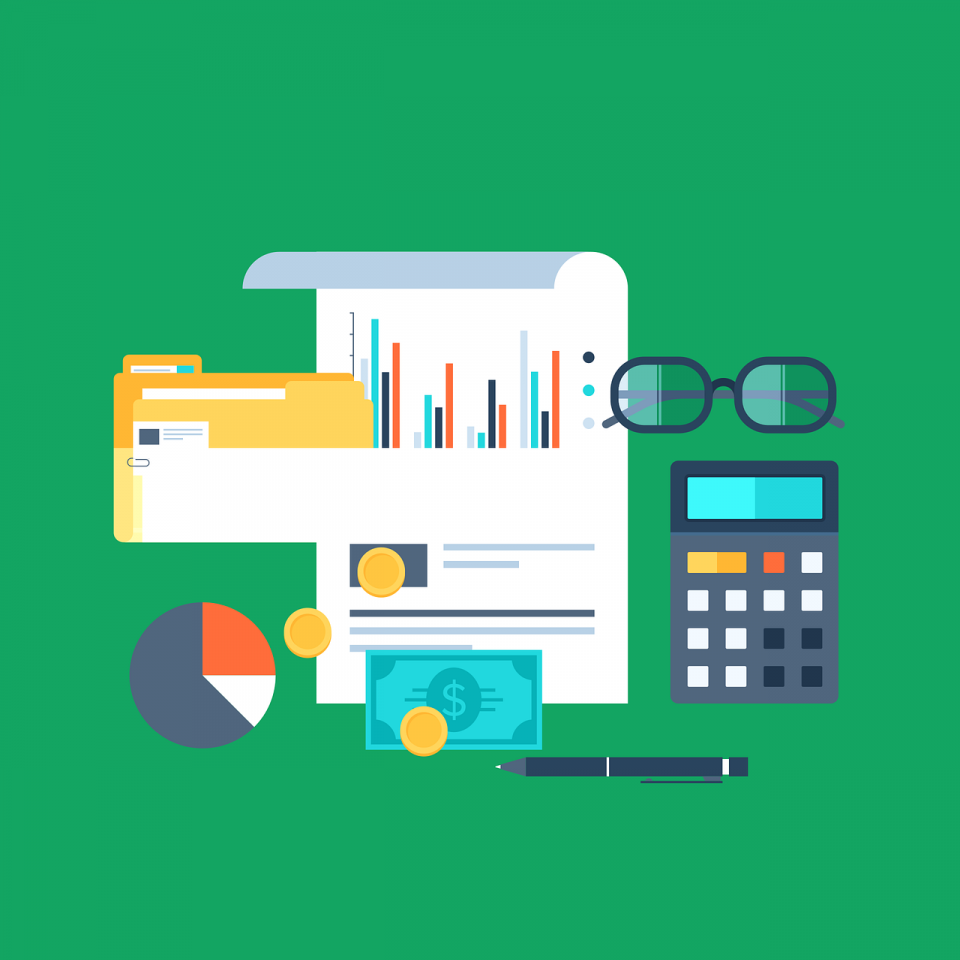This is fairly straightforward to calculate. Let's taLet'slook at an example. Imagine a company is selling a bond with a face value of \$100, and they promise annual coupon payments of \$5.

With this information, we can calculate the rate.

Coupon Rate = (\$100 / \$5) * 100 = 5%

As seen above, the rate is 5%. This rate is annual and does not change throughout the life of the bond, which means that anyone holding this bond will receive \$5 every year that they hold the bond until its maturity date.### Everything You Need To Build Your Accounting Skills

To Help You Thrive in the Most Flexible Job in the World.

## Coupon Rates and Bond Prices

Coupon rates are fixed, but bond prices can be more volatile. The bond price is inversely correlated with the market interest rate: bond prices go down when interest rates go up.

This is because if the market interest rate rises, new bonds will be sold with an interest rate equal to the current market's interest rate, making old bonds worth less money. After all, they pay a lower rate. As a result, bonds adjust their value to make their yield equal to the interest rate.While interest rates are a percentage of the face value of a bond, a bond may be worth more or less than its face value. This is because the change in the bond's price inversely changes the yield the bond offers.

• If market interest rates are higher than the bond's interest rate, the bond's price will be lower.
• If the market rate is lower than the bond's, the bond will sell at a premium.
• If the market rate and the bond's interest rate are the same, the bond will sell at its face value.

## Variations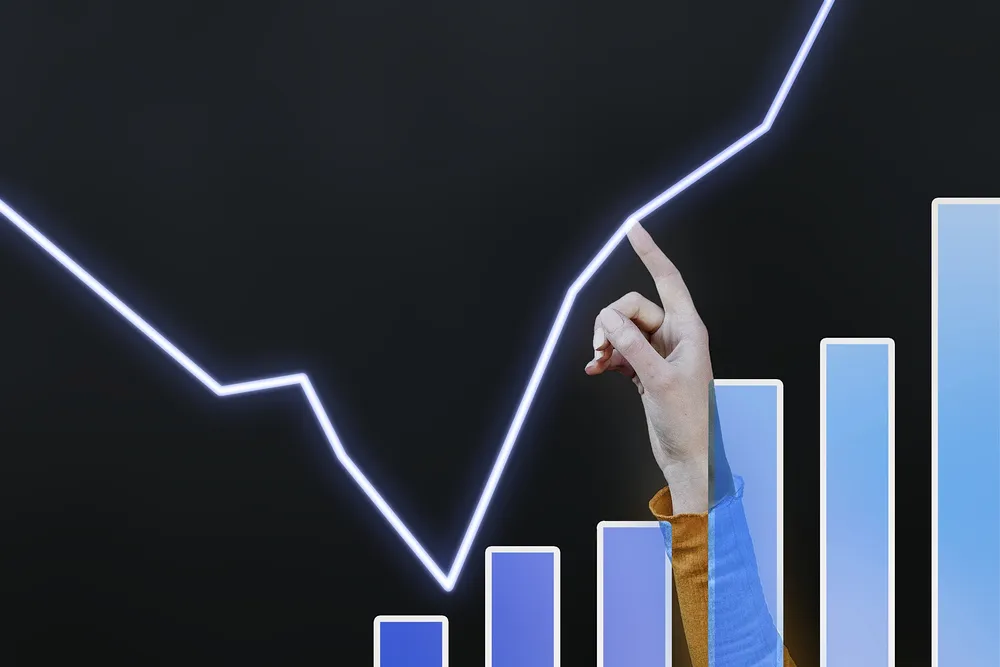Interest rates among bonds may vary for a few reasons, and it's important to understand what drivers a bond's rate might have.

The different reasons are:

• The time value of money
• The credit rating of the issuer
• The market interest rate

All of these have a profound effect on the interest rate of the bond. We go into greater detail on how these affect bonds and their rates below.

Time to maturity can impact interest rates. This has a big impact on the rate at that bonds are sold. The more time there is to maturity, the higher the interest rate on the bond will be. There are multiple reasons for this.

The time value of money is an important factor in any investment, and bonds are no exception. Money is more valuable now rather than later because it can be used to make investments to create more buying power in the future.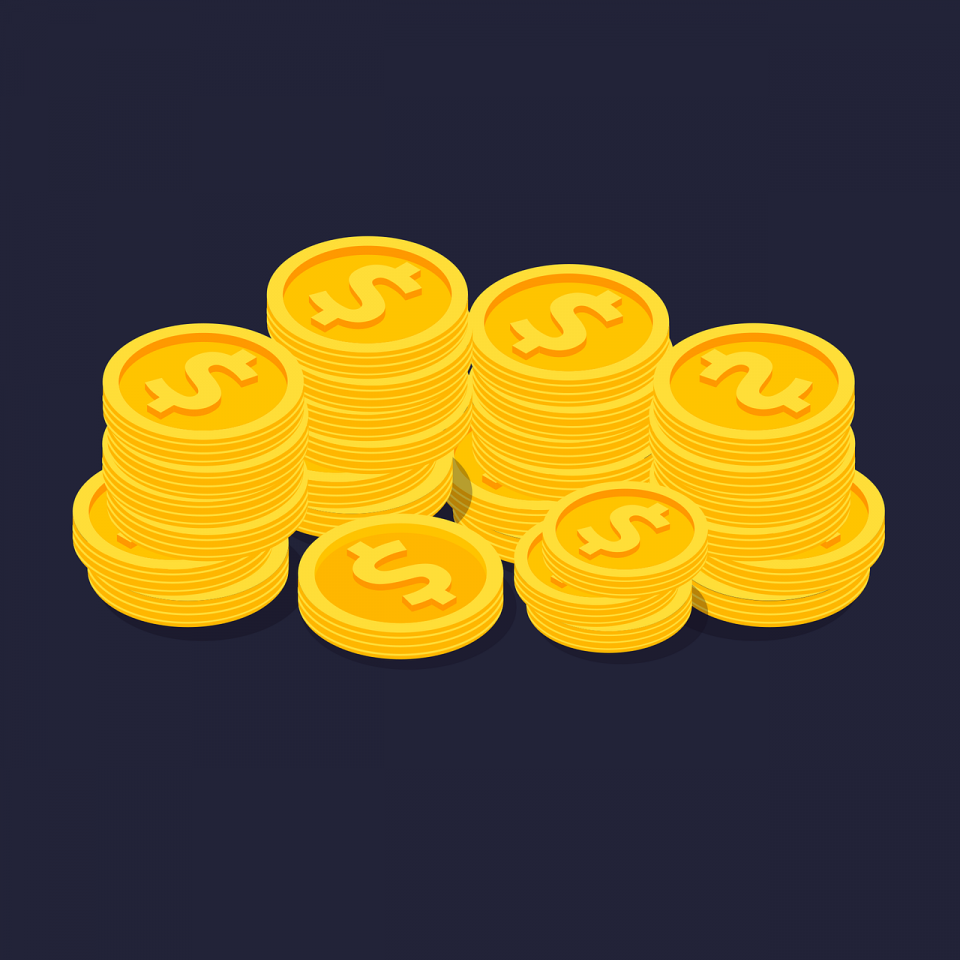In addition, inflation can erode the value of a currency over time, and the same amount of money may have less buying power in the future. Beyond buying power, it is clear that people would rather be able to spend their money when they want instead of having to wait.

The other reason that time is a factor is that there is more risk in waiting for longperiodse to repay a loan. Therefore, there is a higher chance of the debtor defaulting on their debt in a long time rather than a short period.

Simply put, there's more that can go wrong in a longer period rather than a short one.

As you can see, both the time value of money and the risk associated with longer time horizons are the reason that coupon rates vary depending on the amount of time there is to maturity on a bond.

Another important reason interest rates vary is an organization's credit ratings.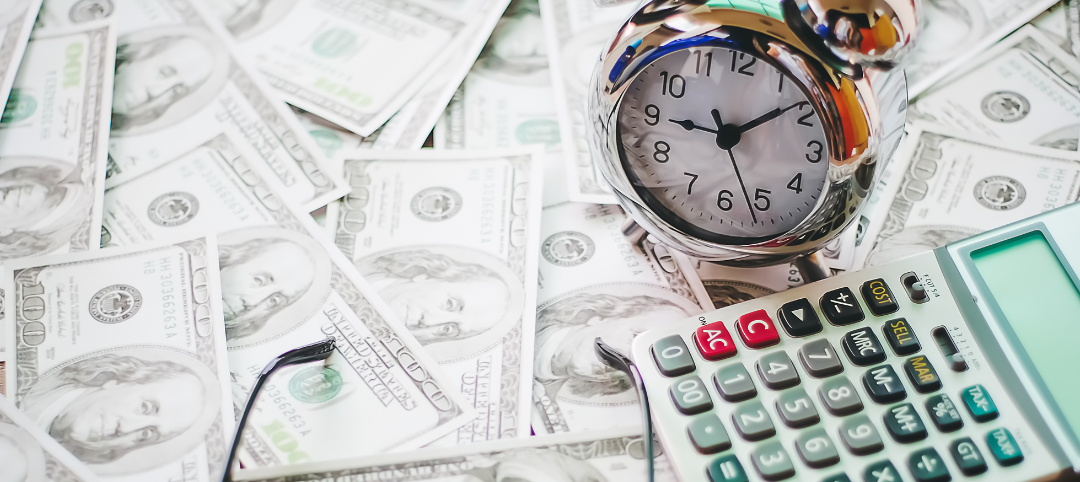The more trustworthy the entity issuing the bonds, the lower the rate on the bond will be. This is because there is less risk for the lender that the debtor will not repay if they are trustworthy.

Look at it this way: organizations will borrow at the interest rate they have to pay to attract borrowers.

If less trustworthy organizations paid the same interest rate as trustworthy organizations, people would only lend to the safe organizations.

Risk greatly affects this rate because organizations will offer the rates they have to attract buyers. This is the cost of borrowing for organizations issuing bonds.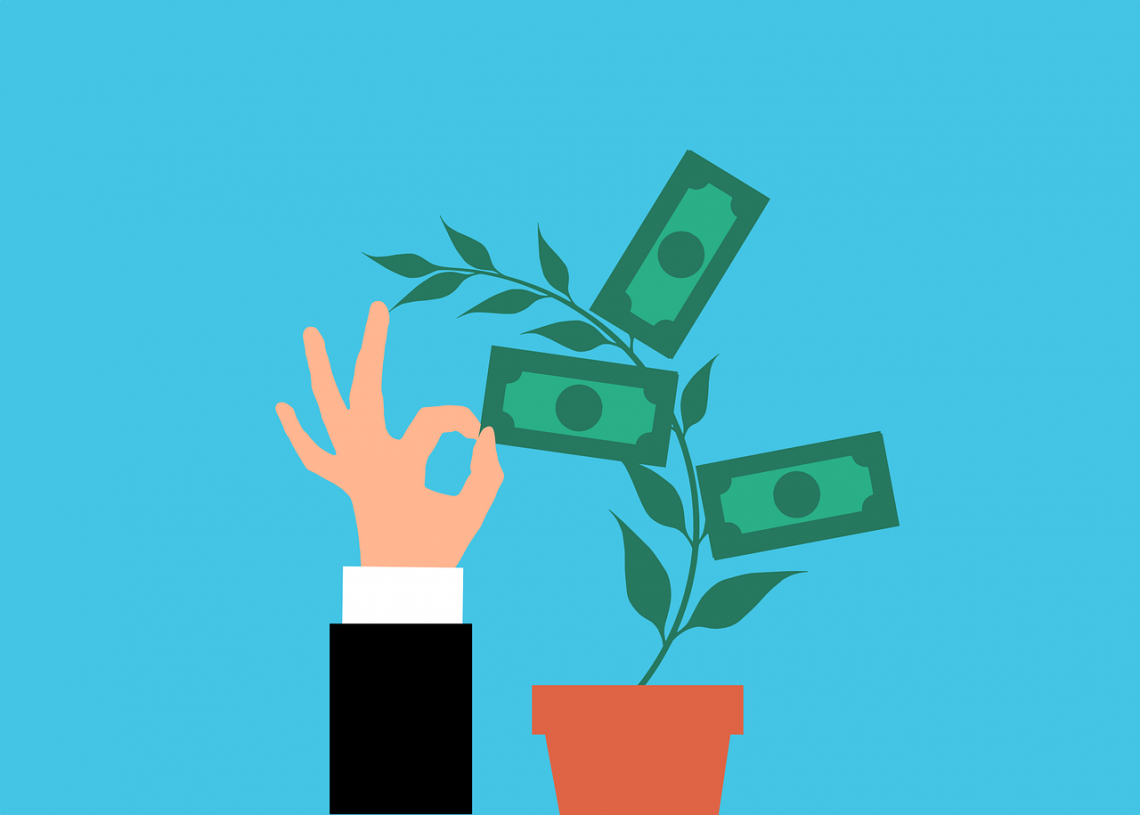Lastly, the market interest rate impacts the coupon rates of new bonds. While rates of old bonds are unchanging, organizations will change their rates as the market interest rate changes.

The central bank sets the interest rate by adjusting the interest rate banks can borrow from the Federal Reserve.

Banks can usually borrow from each other, but sometimes they borrow from the "lender of last resort," otherwise known as the Federal Reserve.

When the Federal Reserve changes the interest rate, it charges banks that borrow from it. Interest rates change from the top down. The banks will adjust their interbank interest rates accordingly, and all other interest rates will also follow.

## Zero-Coupon Bonds

Zero coupon bonds are a rare type of security that doesn't offer any interest rates. Instead, the interest rate on these bonds is 0.

Issuers of zero coupon bonds instead rely heavily on the yield to maturity, discount the sale price, and pay back the principal in full at the bond's maturity date.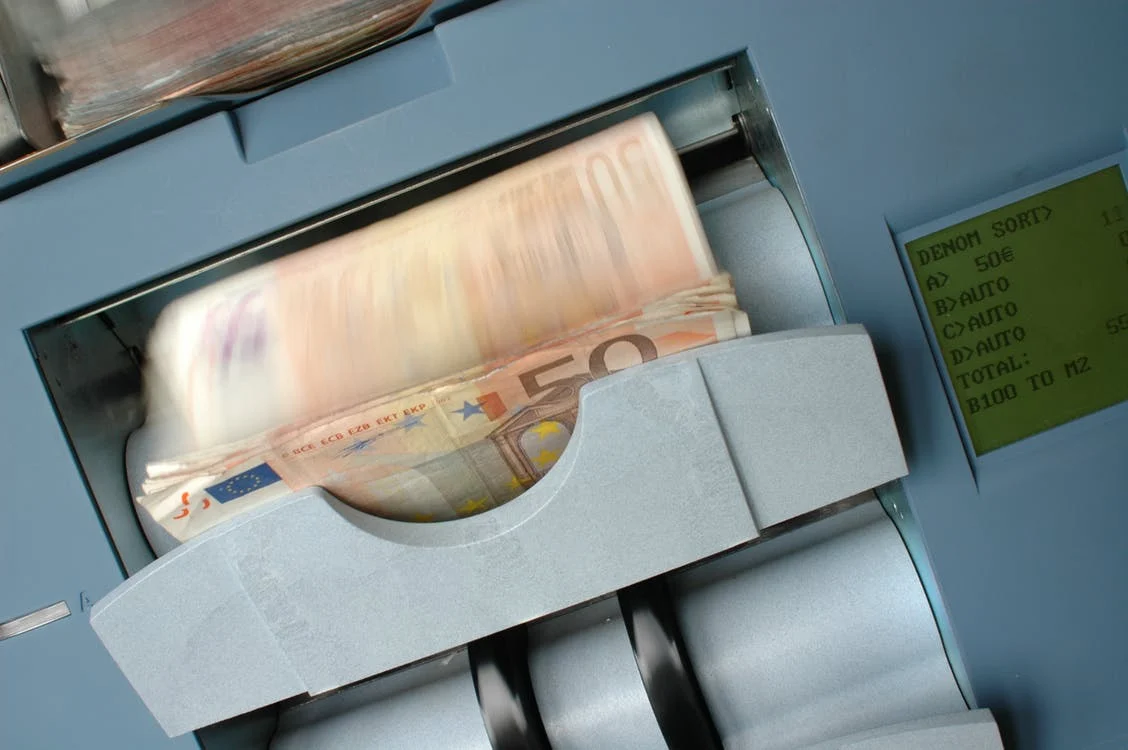While it may not be as desirable because it does not offer periodic coupon payments, these bonds still offer similar returns to other bonds.

For example, imagine a bond worth \$1000withs with a coupon rate of 0 but a yield to maturity of 9%. The bond matures in 10 years. Calculating the maturity is as follows:

Yield to Maturity = 1000 / (1 + 0.09)^10 = 422.41

This bond would sell for only \$422.41, yet it would still return \$1000 upon maturity, giving it a yield to maturity of 9%.

## Coupon Rate vs. Effective Yield

The effective yield is different from the coupon rate. While the coupon rate simply measures the yield, you will receive from the bond at its face value, the effective yield measures the yield a person would receive if they reinvest their coupon payments at the same rate.

The effective yield is found using the coupon rate and can be calculated as follows:

Effective Yield = (1 + c/n)^n - 1

Where,

• c = The coupon rate
• n = The number of payments received annually

This equation may be very important for investors that plan to reinvest their money and want to know how much they can expect to make. This adds another dimension to the interest rate that might be more realistic for investors.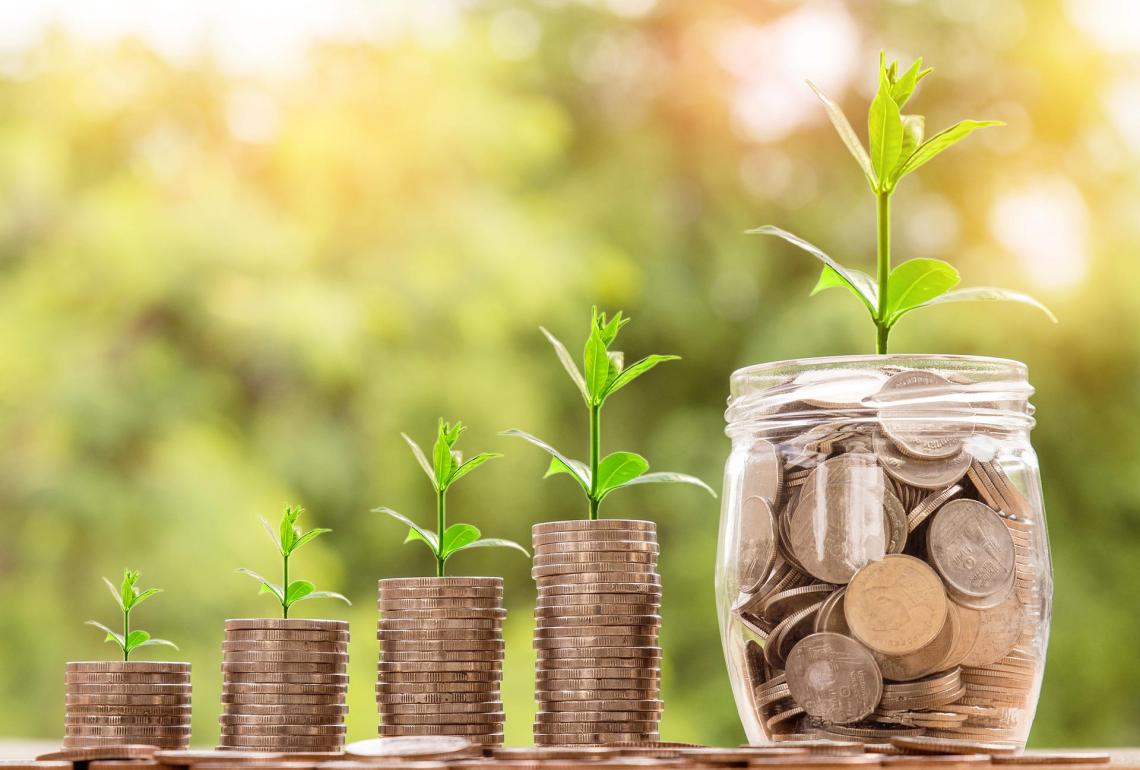## Coupon Rate vs. Yield to Maturity

The coupon rate is the interest payment on the bond, expressed as a percentage of the face value of the bond. The yield to maturity, however, is the internal rate of return (IRR) that a bond offers.

This metric uses a discount rate to discount all the future cash flows from the bond so that its discounted cash flows equal the bond's price. Using the yield to maturity gives investors a better idea of how much profit they can expect.

Discounting the cash flows of the bond also considers the time value of money, which is very important. Because investors know exactly how much money they will make from the bond's future cash flows, they can estimate how much they believe any bond is worth.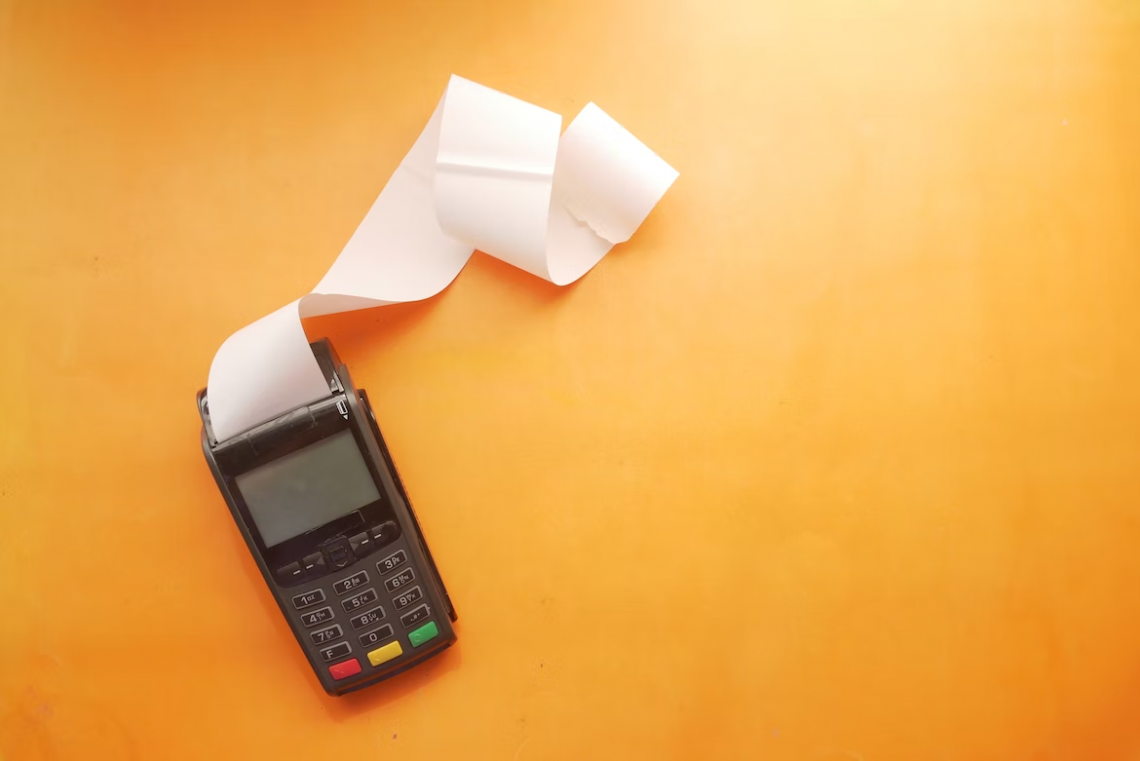The yield to maturity is also different from the interest rate because it does not rely on the face value of the bond.

Bonds may have different prices than their face value. This typically happens when bonds are resold because they will have a shorter time to maturity. In other cases, the market interest rate may have changed and caused the bond price to change.

Using the expected yield to maturity, one can discount the future cash flows and calculate the fair value of a bond that differs from its face value.

Difference
Coupon RateYield of Maturity
• It refers to the sum the issuer pays the bondholder up until the bond's maturity.

• An annual coupon rate is used to pay the interest rate.

• It varies with the interest rate.

• Until the coupon matures, the amount will not change.

• The information on the coupon includes the type of bond paid and the date of issuance.

• Annual Payments / Bond Face Value equals the Coupon Rate, also known as Nominal Yield.

• The coupons are fixed, regardless of the price at which the bond is traded.

• The yield of maturity is the same as the coupon rate.
• The overall return that the investor received up until maturity is referred to as the yield of maturity.

• The current yield shows the annual rate of return.

• The bond's market price is contrasted with the coupon rate to get the current yield.

• Since the market price is always fluctuating, buying bonds at a discount that covers a bigger portion of the purchase price is preferable.
• The yield of maturity determines how much you will receive in the future.

• Yield to Maturity is equal to (C+(F-P)/n)*2/(F+P).

• Prices and yield have an antagonistic relationship.

• Due to the bond being bought at a discount, the maturity yield is greater than the coupon rate.

Researched and Authored by Parth Singhal